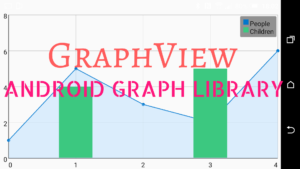# How to show graphs in Android App? [SOLVED] – Android StudioOne might need to show graphs in their app for a lot of reasons. You may want to let the user enter some data and plot the data on a graph and analyse it, or you may want to show some other statistics based on the information from weather, stocks, etc.

In this post I am gonna show you how to achieve this using the library GraphView by Jonas.
The good news is that the library is open source and can be used commercially. The library is distributed under the Apache v2 License(More information here).

Using the library is really easy.

The first step is to add the following dependency to you `GRADLE `file.

```dependencies{
compile 'com.jjoe64:graphview:4.2.1'
}
```

Then just add the GraphView object in your layout using the following code.

```<com.jjoe64.graphview.GraphView
android:layout_width="match_parent"
android:layout_height="200dip"
android:id="@+id/graph" />
```

You need to place the code inside the xml file of your layout. You can change various properties like @id, width, size, etc.

Once you have added to the layout, you can add the data points programmatically inside the activity.
The following code shows one example.

```GraphView graph = (GraphView) findViewById(R.id.graph);
LineGraphSeries<DataPoint> series = new LineGraphSeries<>(new DataPoint[] {
new DataPoint(0, 1),
new DataPoint(1, 5),
new DataPoint(2, 3)
});
```

In the above code, we create an object of `GraphView`, then find it using the @id that you assigned to it earlier.
Then, we create an object ‘`series`‘ of DataPoint, `LineGraphSeries` means that the graph will be a line graph. You can use the following for different types of graphs:
`BarGraphSeries, PointsGraphSeries`

The fun doesn’t end here. You can even mix up different chart types.
The following example illustrates how:

```GraphView graph = (GraphView) findViewById(R.id.graph);
BarGraphSeries<DataPoint> series = new BarGraphSeries<>(new DataPoint[] {
new DataPoint(0, -2),
new DataPoint(1, 5),
new DataPoint(2, 3),
new DataPoint(3, 2),
new DataPoint(4, 6)
});

LineGraphSeries<DataPoint> series2 = new LineGraphSeries<>(new DataPoint[] {
new DataPoint(0, 3),
new DataPoint(1, 3),
new DataPoint(2, 6),
new DataPoint(3, 2),
new DataPoint(4, 5)
});
```

Reference

You can even enable scrolling and zooming:

```// first series is a line
DataPoint[] points = new DataPoint;
for (int i = 0; i < points.length; i++) {
points[i] = new DataPoint(i, Math.sin(i*0.5) * 20*(Math.random()*10+1));
}
LineGraphSeries<DataPoint> series = new LineGraphSeries<>(points);

// set manual X bounds
graph.getViewport().setYAxisBoundsManual(true);
graph.getViewport().setMinY(-150);
graph.getViewport().setMaxY(150);

graph.getViewport().setXAxisBoundsManual(true);
graph.getViewport().setMinX(4);
graph.getViewport().setMaxX(80);

// enable scaling and scrolling
graph.getViewport().setScalable(true);
graph.getViewport().setScalableY(true);